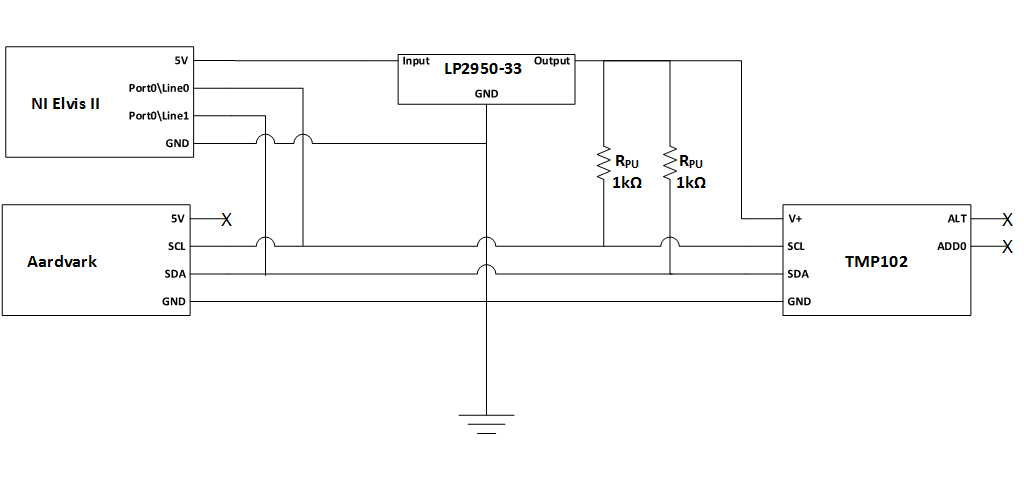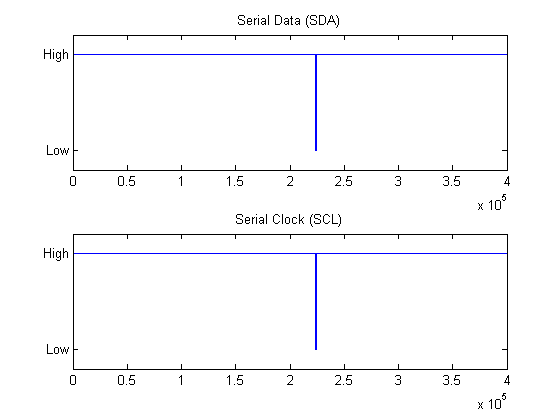## Communicate with I2C devices and analyze bus signals using digital IO

MATLAB® is able to communicate with instruments and devices at the protocol layer as well as the physical layer. This example uses the I2C feature of the Instrument Control Toolbox to communicate with a TMP102 temperature sensor, and simultaneously analyze the physical layer I2C bus communications using the clocked digital IO feature of the Data Acquisition Toolbox.

This example requires the Data Acquisition Toolbox™ and Instrument Control Toolbox™

### Hardware configuration and schematic

• Any Session Based Interface supported National Instruments™ DAQ device with clocked DIO channels can be used (e.g. NI Elvis II)

• TotalPhase Aardvark I2C/SPI Host Adaptor

• TMP102 Digital Temperature Sensor with two-wire serial interface

The TMP102 requires a 3.3V supply. We used a linear LDO (LP2950-33) to generate the 3.3V supply from the DAQ device's 5V supply line.

Alternative options include:

• Use an external power supply.

• Use an Analog Output channel from your DAQ device.### Connect to TMP102 sensor using I2C host adaptor and read temperature data

Hook up the sensor and verify that you can communicate with it using the I2C object from the Instrument Control Toolbox.

```aa = instrhwinfo('i2c', 'aardvark'); % Get information about connected I2C hosts tmp102 = i2c('aardvark',0,hex2dec('48')); % Create an I2C object to connect to the TMP102 tmp102.PullupResistors = 'both'; % Use host adaptor pull-up resistors fopen(tmp102); % Open the connection data8 = fread(tmp102, 2, 'uint8'); % Read 2 byte data % One LSB equals 0.0625 deg. C temperature = ... (double(bitshift(int16(data8(1)), 4)) +... double(bitshift(int16(data8(2)), -4))) * 0.0625; % Refer to TMP102 data sheet to calculate temperature from received data fprintf('The temperature recorded by the TMP102 sensor is: %s deg. C\n',num2str(temperature)); fclose(tmp102); ```
```The temperature recorded by the TMP102 sensor is: 27.625 deg. C ```

### Acquire the corresponding I2C physical layer signals using a DAQ device

Use oversampled clocked digital channels to acquire and analyze the physical layer communications on the I2C bus. In our setup, the NI Elvis II had Device ID `Dev4`.

Acquire SDA data on port 0, line 0 of your DAQ device. Acquire SCL data on port 0, line 1 of your DAQ device.

```s = daq.createSession('ni'); addDigitalChannel(s,'Dev4', 'port0\line0', 'InputOnly'); % sda addDigitalChannel(s,'Dev4', 'port0\line1', 'InputOnly'); % scl ```

### Generate a clock signal for use with your digital subsystem

Digital subsystems on NI DAQ devices do not have their own clock; they must share a clock with the analog subsystem or receive a clock from an external subsystem. In this example, we shall use a Counter Output Pulse Generation channel to generate a 50% duty cycle clock at a frequency of 1,000,000 Hz, and set the session rate to match.

```pgChan = addCounterOutputChannel(s,'Dev4', 1, 'PulseGeneration'); s.Rate = 1e6; pgChan.Frequency = s.Rate; ```

The clock is generated on the 'pgChan.Terminal' pin, allowing you to synchronize with other devices or view the clock on an oscilloscope. In this example, we import the counter output pulse signal back into the session to be used as a clock signal.

```disp(pgChan.Terminal); addClockConnection(s,'External',['Dev4/' pgChan.Terminal],'ScanClock'); ```
```PFI13 ```

### Add a listener to the session object.

Create a listener to collect acquired data to a global variable `myData` while a session is running in background mode.

```type saveData.m global myData; addlistener(s,'DataAvailable', @saveData); ```
```function saveData(src, evt) global myData myData = [myData; evt.Data]; end ```

### Acquire the I2C signals using clocked digital channels

Set the session to run in continuous mode until stopped.

```s.IsContinuous = true; ```

Run the session in background mode accumulating the results in a global variable 'myData'. Accumulate acquired data with a callback function which simply appends acquired data to a column based global array variable.

Start the session in background mode accumulating acquired data on the SDA and SCL digital lines to the global variable `myData`.

• Start the session in background mode

• Start the I2C operations

• Stop the session when done

```myData = []; s.startBackground; fopen(tmp102); data8 = fread(tmp102, 2, 'uint8'); % One LSB equals 0.0625 deg. C temperature = (double(bitshift(int16(data8(1)), 4)) +... double(bitshift(int16(data8(2)), -4))) * 0.0625; fclose(tmp102); pause(0.1); s.stop(); ```
```Warning: Trigger and Clock Connections will not affect counter output channels. ```

Plot the raw data to see the acquired signals. Notice that lines are held high during idle periods. In next section will show you how to find the start/stop condition bits and use them to isolate areas of interest in the I2C communication.

```figure('Name', 'Raw Data'); subplot(2,1,1); plot(myData(:,1)); ylim([-0.2, 1.2]); ax = gca; ax.YTick = [0,1]; ax.YTickLabel = {'Low','High'}; title('Serial Data (SDA)'); subplot(2,1,2); plot(myData(:,2)); ylim([-0.2, 1.2]); ax = gca; ax.YTick = [0,1]; ax.YTickLabel = {'Low','High'}; title('Serial Clock (SCL)'); ```### Analyze the I2C physical layer bus communications

Extract I2C physical layer signals on the SDA and SCL lines

```sda = myData(:,1)'; scl = myData(:,2)'; ```

Find all rising and falling clock edges

```sclFlips = xor(scl(1:end-1), scl(2:end)); sclFlips = [1 sclFlips 1]; sclFlipIndexes = find(sclFlips==1); ```

Calculate the clock periods from the clock indices

```sclFlipPeriods = sclFlipIndexes(1:end)-[1 sclFlipIndexes(1:end-1)]; ```

Through inspection, we assume idle periods are periods having SCL high for longer than 100us. Since rate = 1MS/s, each sample represents 1 us.

idlePeriodIndices: This variable allows us to maneuver between periods of activity within the I2C communication.

```idlePeriodIndices = find(sclFlipPeriods>100); ```

Zoom into the first period of activity on the I2C bus. For ease of viewing include 30 samples of idle activity to the front and end of each plot.

```range1 = sclFlipIndexes(idlePeriodIndices(1)) - 30 : sclFlipIndexes(idlePeriodIndices(2) - 1) + 30; figure('Name', 'I2C Communication Data'); subplot(2,1,1); plot(sda(range1)); ylim([-0.2, 1.2]); ax = gca; ax.YTick = [0,1]; ax.YTickLabel = {'Low','High'}; title('Serial Data (SDA)'); subplot(2,1,2); plot(scl(range1)); ylim([-0.2, 1.2]); ax = gca; ax.YTick = [0,1]; ax.YTickLabel = {'Low','High'}; title('Serial Clock (SCL)'); ```### Analyze bus performance metrics.

As a simple example we will analyze start and stop condition metrics, and I2C bit rate calculation.

• Start condition duration shall be defined as the time it took for SCL to go low after SDA goes low.

• Stop condition duration shall be defined as the time it took for SDA to go high after SCL goes high.

• Bit rate will be calculated by taking the inverse of the time between 2 rising clock edges.

START CONDITION: first SDA low, then SCL low

```sclLowIndex = sclFlipIndexes(idlePeriodIndices(1)); sdaLowIndex = find(sda(1:sclLowIndex)==1, 1, 'last') + 1; % +1, flip is next value after last high startConditionDuration = (sclLowIndex - sdaLowIndex) * 1/s.Rate; fprintf('sda: %s\n', sprintf('%d ', sda(sdaLowIndex-1:sclLowIndex))); % Indexes point to next change, hence sclLowIndex includes flip to low fprintf('scl: %s\n', sprintf('%d ', scl(sdaLowIndex-1:sclLowIndex))); % subtract 1 from sdaLowIndex to see sda value prior to flip fprintf('Start condition duration: %d sec.\n\n', startConditionDuration); % count 5 pulses, 5 us. ```
```sda: 1 0 0 0 0 0 0 scl: 1 1 1 1 1 1 0 Start condition duration: 5.000000e-06 sec. ```

STOP CONDITION: first SCL high, then SDA high

```% flip prior to going into idle is the one we want sclHighIndex = sclFlipIndexes(idlePeriodIndices(2)-1); sdaHighIndex = find(sda(sclHighIndex:end)==1, 1, 'first') + sclHighIndex - 1; stopConditionDuration = (sdaHighIndex - sclHighIndex) * 1/s.Rate; fprintf('sda: %s\n', sprintf('%d ',sda(sclHighIndex-1:sdaHighIndex))); fprintf('scl: %s\n', sprintf('%d ',scl(sclHighIndex-1:sdaHighIndex))); fprintf('Stop condition duration: %d sec.\n\n', stopConditionDuration); ```
```sda: 0 0 0 0 0 0 1 scl: 0 1 1 1 1 1 1 Stop condition duration: 5.000000e-06 sec. ```

BIT RATE: Inverse of time between 2 rising edges on the SCL line

```startConditionIndex = idlePeriodIndices(1); firstRisingClockIndex = startConditionIndex + 2; secondRisingClockIndex = firstRisingClockIndex + 2; clockPeriodInSamples = sclFlipIndexes(secondRisingClockIndex) - sclFlipIndexes(firstRisingClockIndex); clockPeriodInSeconds = clockPeriodInSamples * 1/s.Rate; bitRate = 1/clockPeriodInSeconds; fprintf('DAQ calculated bit rate = %d; Actual I2C object bit rate = %dKHz\n', ... bitRate,... tmp102.BitRate); ```
```DAQ calculated bit rate = 1.000000e+05; Actual I2C object bit rate = 100KHz ```

### Find the bit stream by sampling on the rising edges.

The `sclFlipIndexes` vector was created using XOR and hence contains both rising and falling edges. Start with a rising edge and use a step of two to skip falling edges.

```% idlePeriodIndices(1)+1 is first rising clock edge after start condition. % Use a step of two to skip falling edges and only look at rising edges. % idlePeriodIndices(2)-1 is the index of the rising edge of the stop condition. % idlePeriodIndices(2)-3 is the last rising clock edge in the bit stream to be % decoded. bitStream = sda(sclFlipIndexes(idlePeriodIndices(1)+1:2:idlePeriodIndices(2)-3)); fprintf('Raw bit stream extracted from I2C physical layer signal: %s\n\n', sprintf('%d ', bitStream)); ```
```Raw bit stream extracted from I2C physical layer signal: 1 0 0 1 0 0 0 1 0 0 0 0 1 1 0 1 1 0 1 0 1 0 0 0 0 0 1 ```

### Decode the acquired bit stream

```ADR_RW = {'W', 'R'}; ACK_NACK = {'ACK', 'NACK'}; address = bitStream(1:7); % 7 bit address fprintf('\nDecoded Address: %d%d%d%d%d%d%d(0x%s) %d(%s) %d(%s)\n', ... address,... binaryVectorToHex(address),... bitStream(8),... ADR_RW{bitStream(8)+1},... bitStream(9),... ACK_NACK{bitStream(9)+1}); for iData = 0:1 startBit = 10 + iData*9; endBit = startBit + 7; ackBit = endBit + 1; data = bitStream(startBit:endBit); fprintf('Decoded Data%d: %s(0x%s) %d(%s)\n', ... iData+1,... sprintf('%d', data),... binaryVectorToHex(data),... bitStream(ackBit),... ACK_NACK{bitStream(ackBit)+1}); end ```
```Decoded Address: 1001000(0x48) 1(R) 0(ACK) Decoded Data1: 00011011(0x1B) 0(ACK) Decoded Data2: 10100000(0xA0) 1(NACK) ```

### Verify that the data we decoded using DAQ matches the data we read using ICT

Two `uint8` bytes were 'fread' from the I2C bus into variable `data8`... The hex conversion of these values should match the results of the bus decode shown above.

```fprintf('Data acquired from I2C object: 0x%s\n', dec2hex(data8)'); fprintf('Temperature: %2.2f deg. C\n\n', temperature); ```
```Data acquired from I2C object: 0x1BA0 Temperature: 27.63 deg. C ```
##### Support